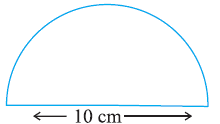# Ex.11.3 Q7 Perimeter and Area - NCERT Maths Class 7

Go back to  'Ex.11.3'

## Question

Find the perimeter of the adjoining figure, which is a semicircle including its diameterVideo Solution
Perimeter And Area
Ex 11.3 | Question 7

## Text Solution

What is known?

Diameter of the semicircle.

What is unknown?

The perimeter of the semicircle including its diameter.

Reasoning:

To find the perimeter of the given figure, add the circumference or perimeter of the semi-circle and the diameter of the given figure.

Steps:

Given, diameter $$= 10 \rm\,cm$$

Radius \begin{align}=\frac{{10}}{2}\end{align}= 5 \rm\,cm

Perimeter of the given figure $$=$$ Circumference of semi $$-$$ circle $$+$$ diameter

\begin{align}&= \frac{{2\pi r}}{2} + {\text{D}} \\{\text{ }}&= \pi r + {\text{D}} \\&= \frac{{22}}{7} \times 5 + 5 \\&= \frac{{110}}{7} + 5 \\&= 25.71\;\rm cm\end{align}

Thus, the perimeter of the given figure is $$25.71\rm\, cm.$$

Learn from the best math teachers and top your exams

• Live one on one classroom and doubt clearing
• Practice worksheets in and after class for conceptual clarity
• Personalized curriculum to keep up with school